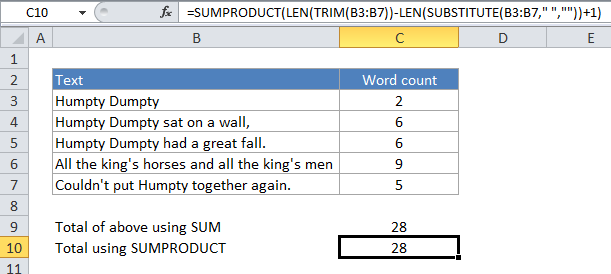# How to count total words in a range in Excel

If you want to count the total words in a range of cells, you can do with a formula that uses LEN and SUBSTITUTE, along with the SUMPRODUCT function.

## Formula

`=SUMPRODUCT(LEN(TRIM(range))-LEN(SUBSTITUTE(range," ",""))+1)`Note: The formula inside SUMPRODUCT will return 1 even if a cell is empty. If you need to guard against this problem, you can add another array to SUMPRODUCT as below. The double hyphen coerces the result to 1’s and 0’s. We use TRIM again to make sure we don’t count cells that have one or more spaces.

`=SUMPRODUCT((LEN(TRIM(B3:B7))-LEN(SUBSTITUTE(B3:B7," ",""))+1),--(TRIM(B3:B7)<>""))`

## Explanation

In the example above, we are using:

`=SUMPRODUCT(LEN(TRIM(B3:B7))-LEN(SUBSTITUTE(B3:B7," ",""))+1)`

### Here’s how the formula works:

For each cell in the range, SUBSTITUTE removes all spaces from the text, then LEN calculates the length of the text without spaces. This number is then subtracted from the length of the text with spaces, and the number 1 is added to the final result, since the number of words is the number of spaces + 1. We’re using TRIM to remove any extra spaces between words, or at the beginning or end of the text.

Also See:   Remove first character in a cell in Excel

The result of all this calculation is a list of items, where there is one item per cell in the range, and each item a number based on the calculation above. In other words, we have a list of word counts, with one word count per cell.

SUMPRODUCT then sums this list and returns a total for all cells in the range.

Also See:   Count cells not equal to many things in Excel